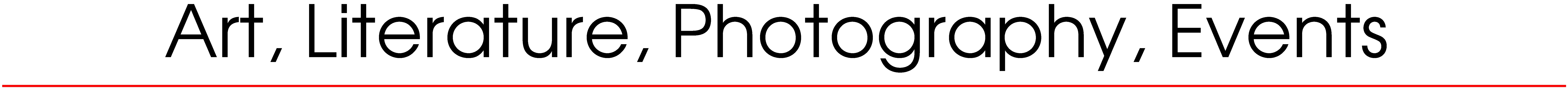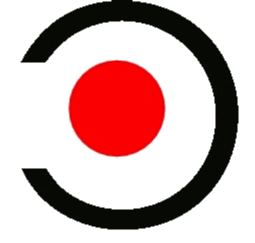Numbers as Words as Found Art When text spelled the number so that it could be read rather than merely recognized as digits. Eighteen • Fifty • Ninty • One • Six • Sixty-Three • Ten • Three • Twenty • Twenty-One • Twenty-Three • Two • Two-Hundred • Zero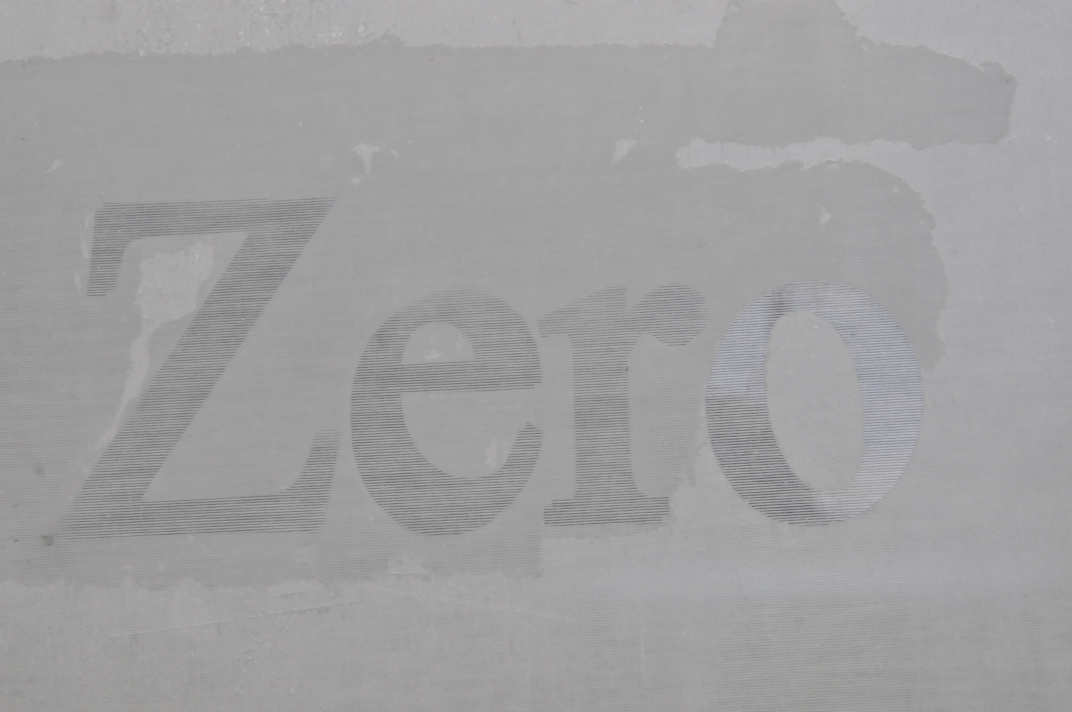Zero, Number as a Word, as Found Art0 (zero) is both a number and the numerical digit used to represent that number in numerals. The number 0 fulfills a central role in mathematics as the additive identity of the integers, real numbers, and many other algebraic structures. [from wikipedia]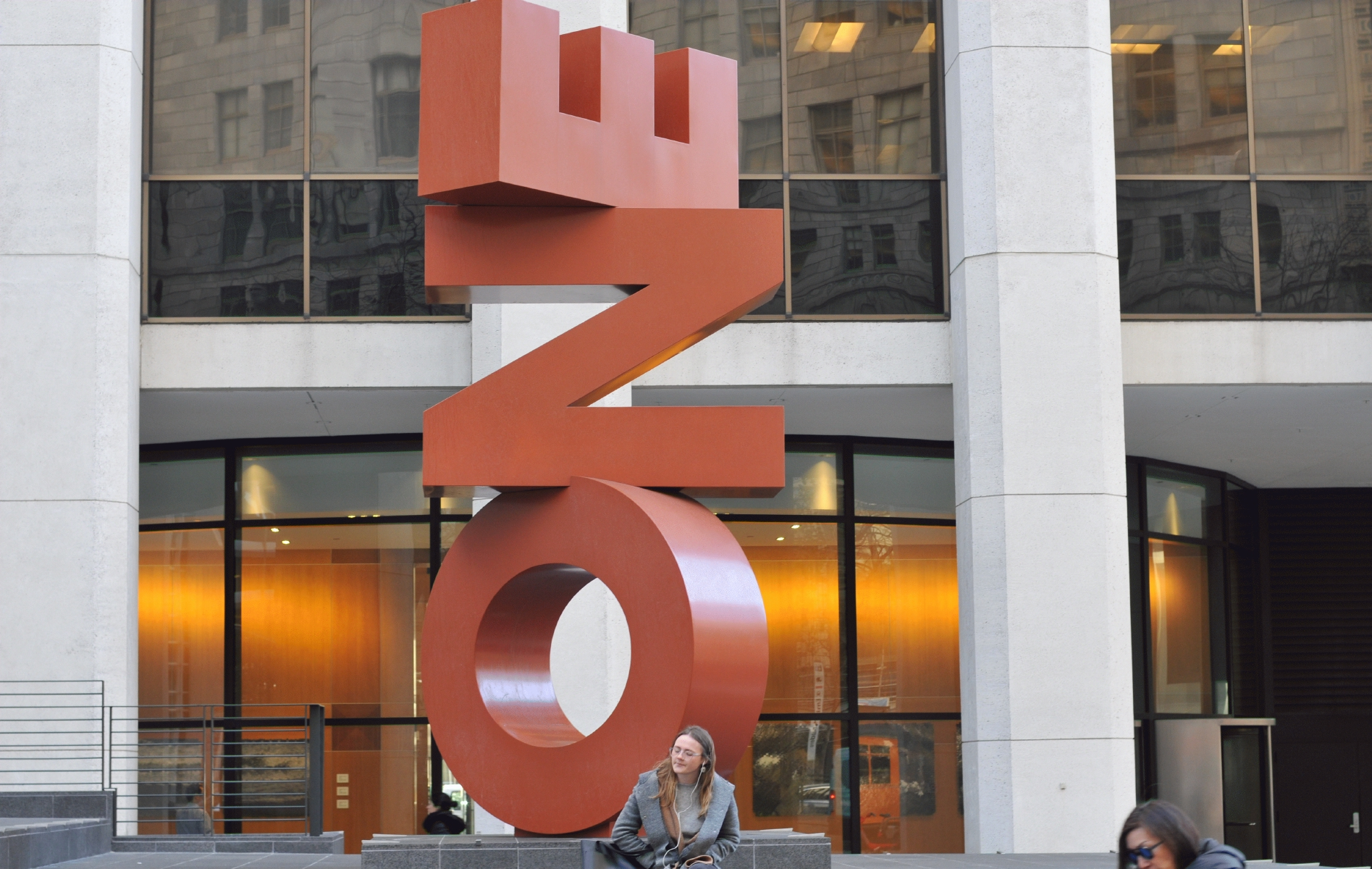One, Number as a Word, as Found Art1 (one, also called unit, unity, and (multiplicative) identity) is a number, numeral, and glyph. It represents a single entity, the unit of counting or measurement. For example, a line segment of unit length is a line segment of length 1. It is also the first of the infinite sequence of natural numbers, followed by 2.[from wikipedia]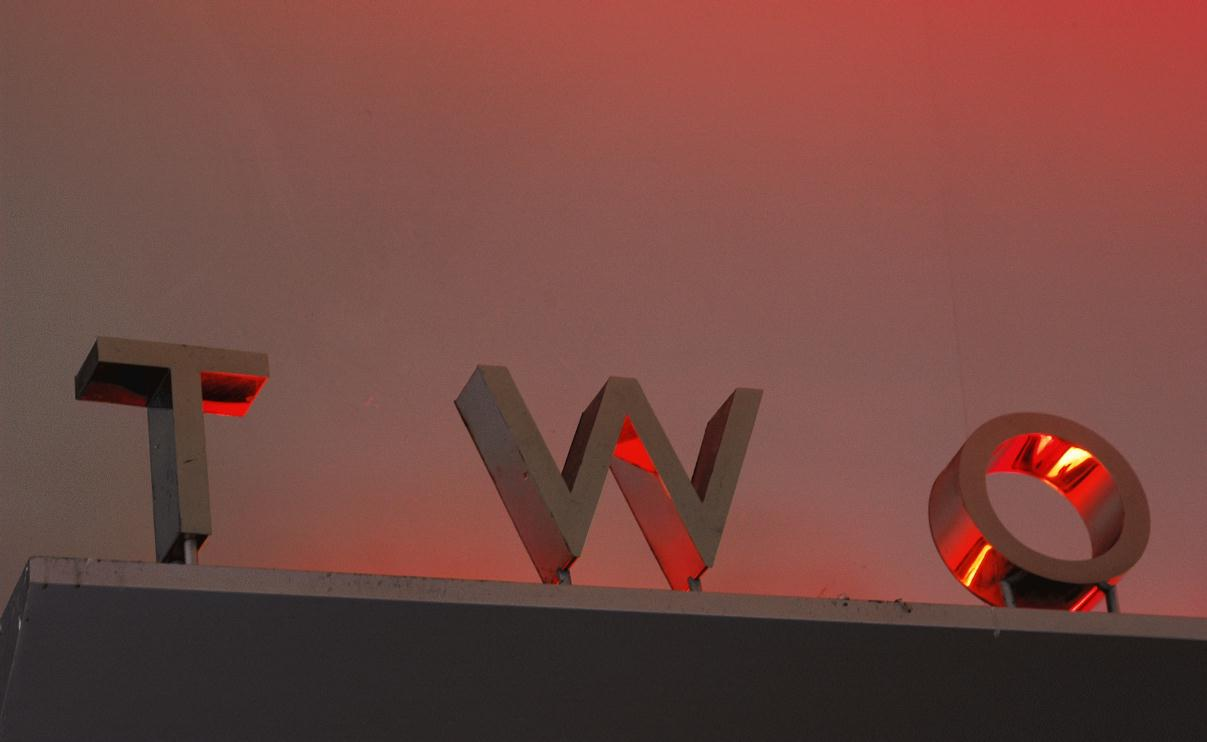Two, Number as a Word, as Found Art2 (two) is a number, numeral, and glyph. It is the natural number following 1 and preceding 3.[from wikipedia]Three, Number as a Word, as Found Art3 is a number, numeral, and glyph. [from wikipedia]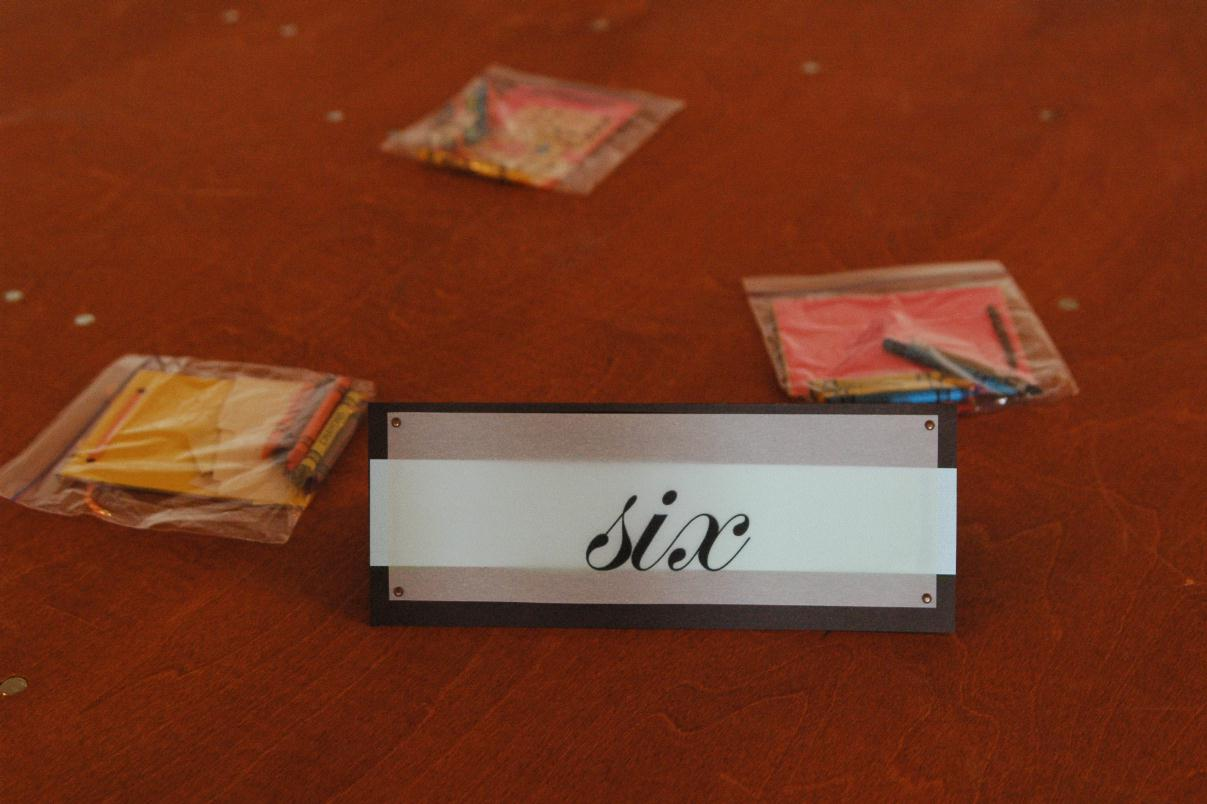Six, Number as a Word, as Found Art6 is a number, numeral, and glyph. [from wikipedia]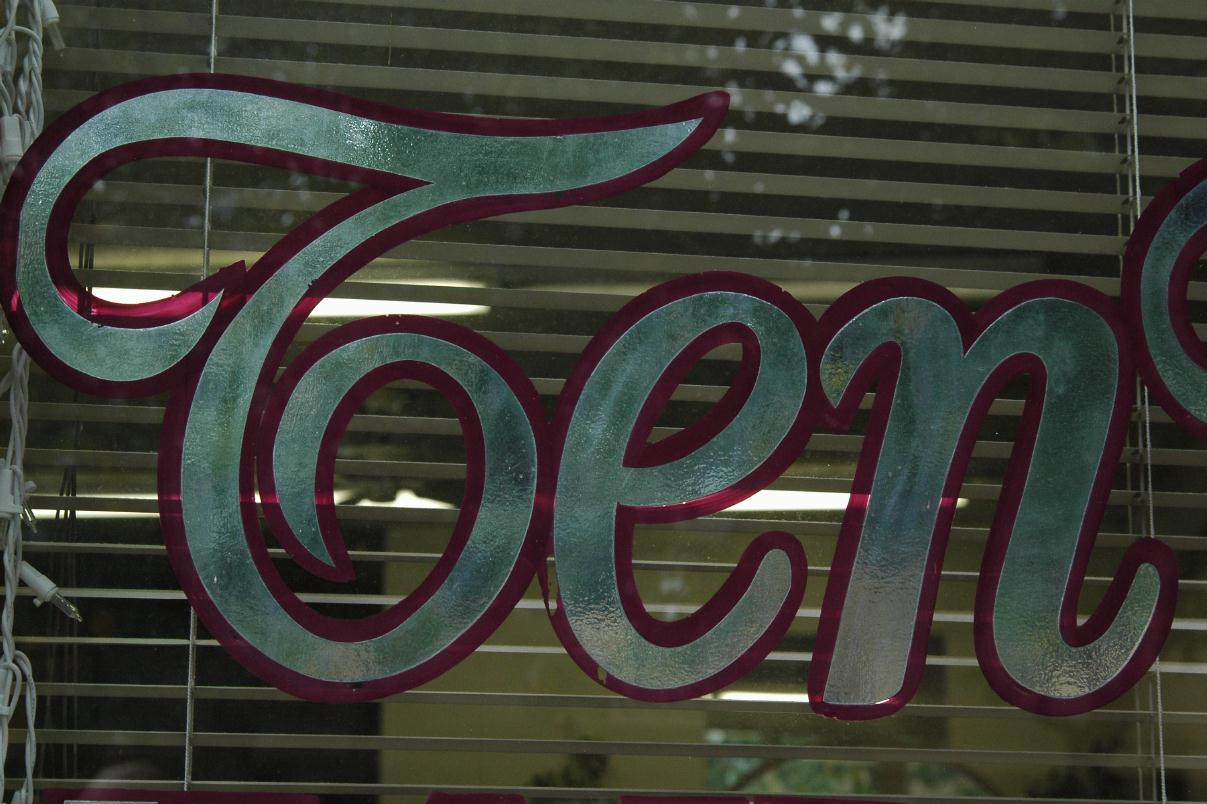Ten, Number as a Word, as Found Art10 (ten) is an even natural number following 9 and preceding 11. Ten is the base of the decimal numeral system, by far the most common system of denoting numbers in both spoken and written language. The reason for the choice of ten is assumed to be that humans have ten fingers (digits). [from wikipedia]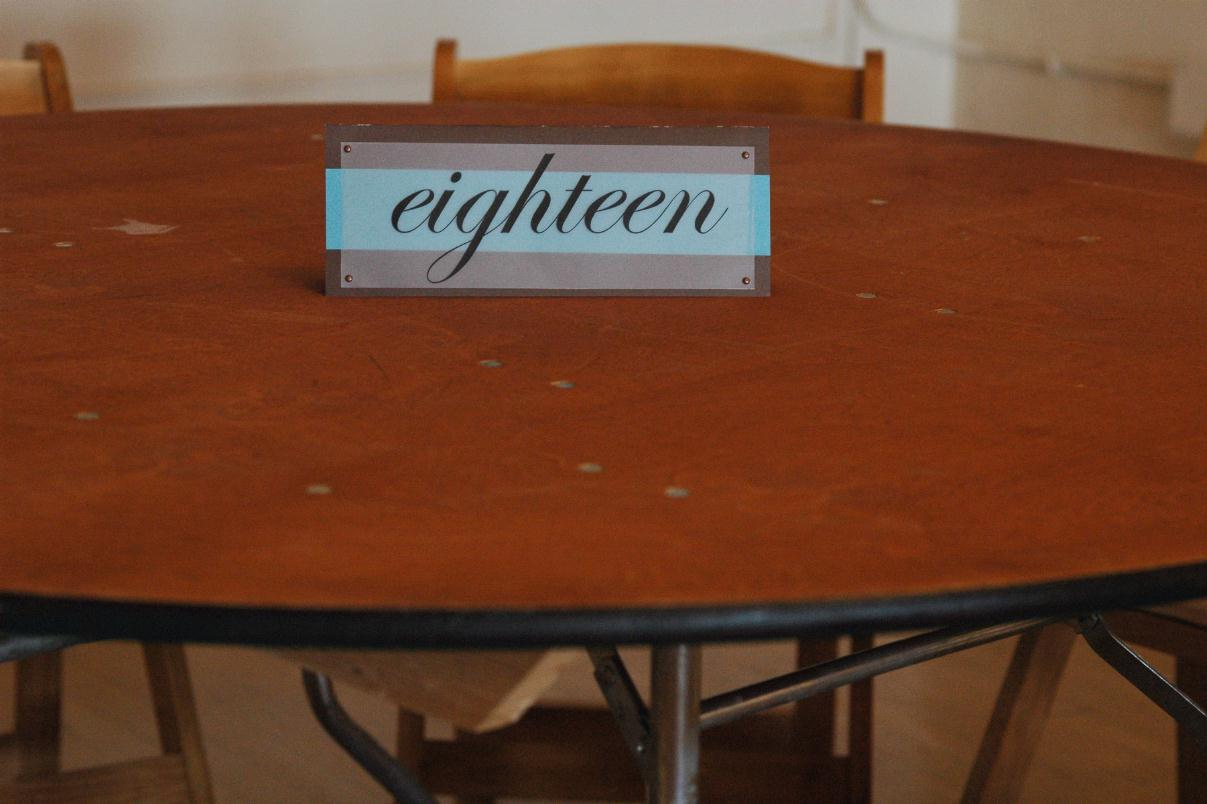Eighteen, Number as a Word, as Found Art18 (eighteen) is the natural number following 17 and preceding 19. In English speech, the numbers 18 and 80 are sometimes confused, as they sound very similar. [from wikipedia]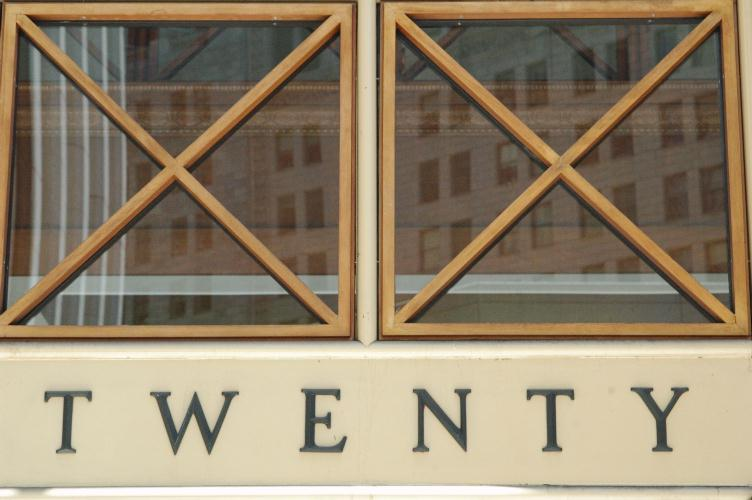Twenty, Number as a Word, as Found Art20 (twenty) is the natural number following 19 and preceding 21. A group of twenty units may also be referred to as a score.[from wikipedia]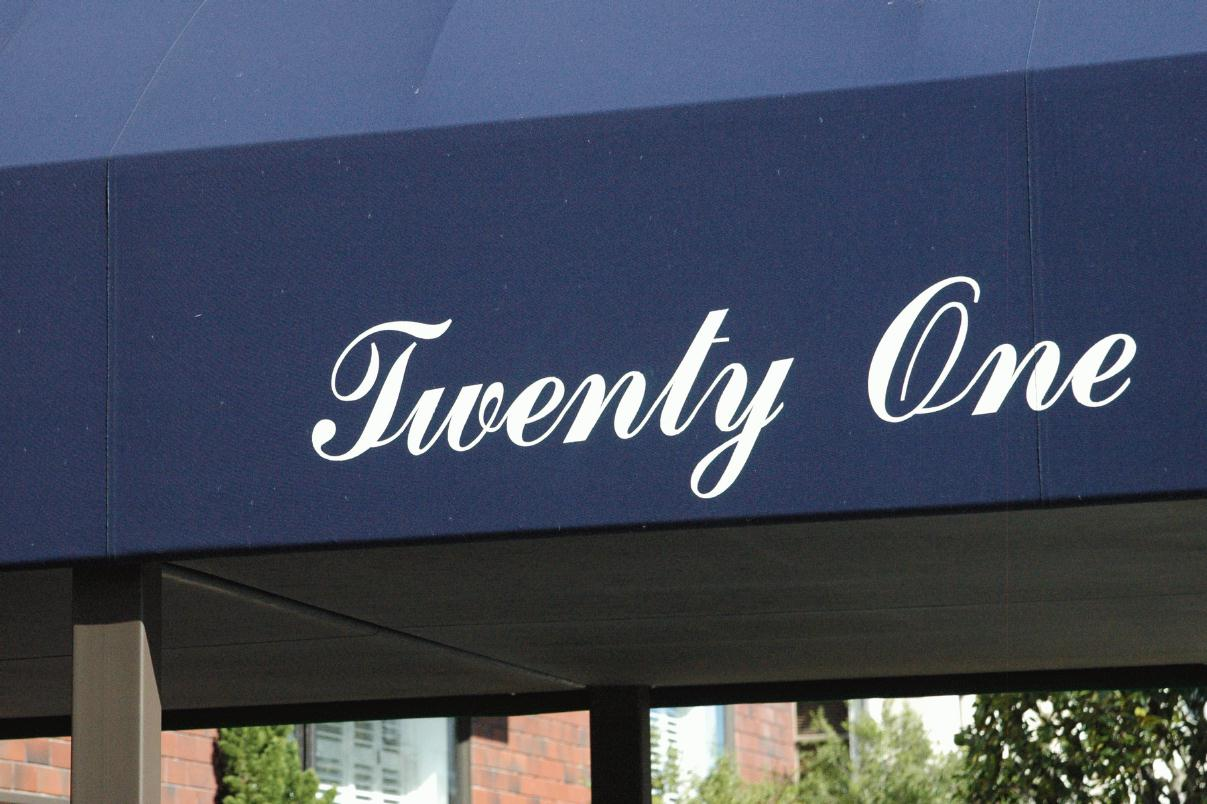Twenty-One, Number as a Word, as Found Art21 (twenty-one) is the natural number following 20 and preceding 22. [from wikipedia]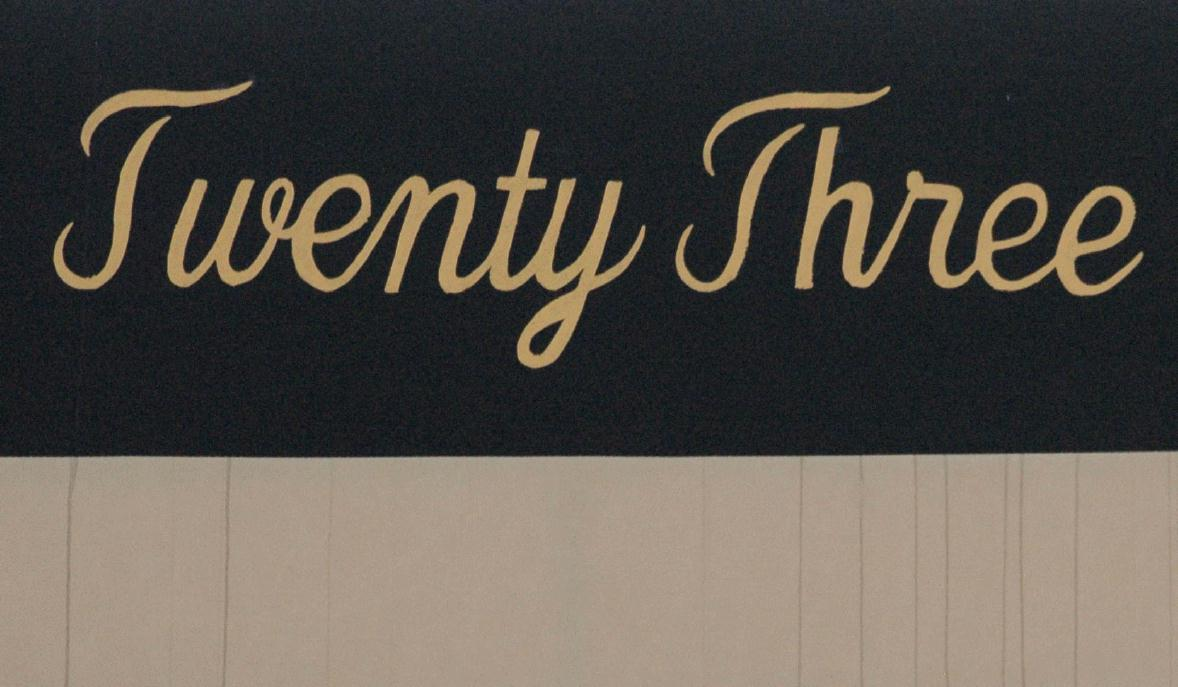Twenty-Three, Number as a Word, as Found Art23 (twenty-three) is the natural number following 22 and preceding 24. [from wikipedia]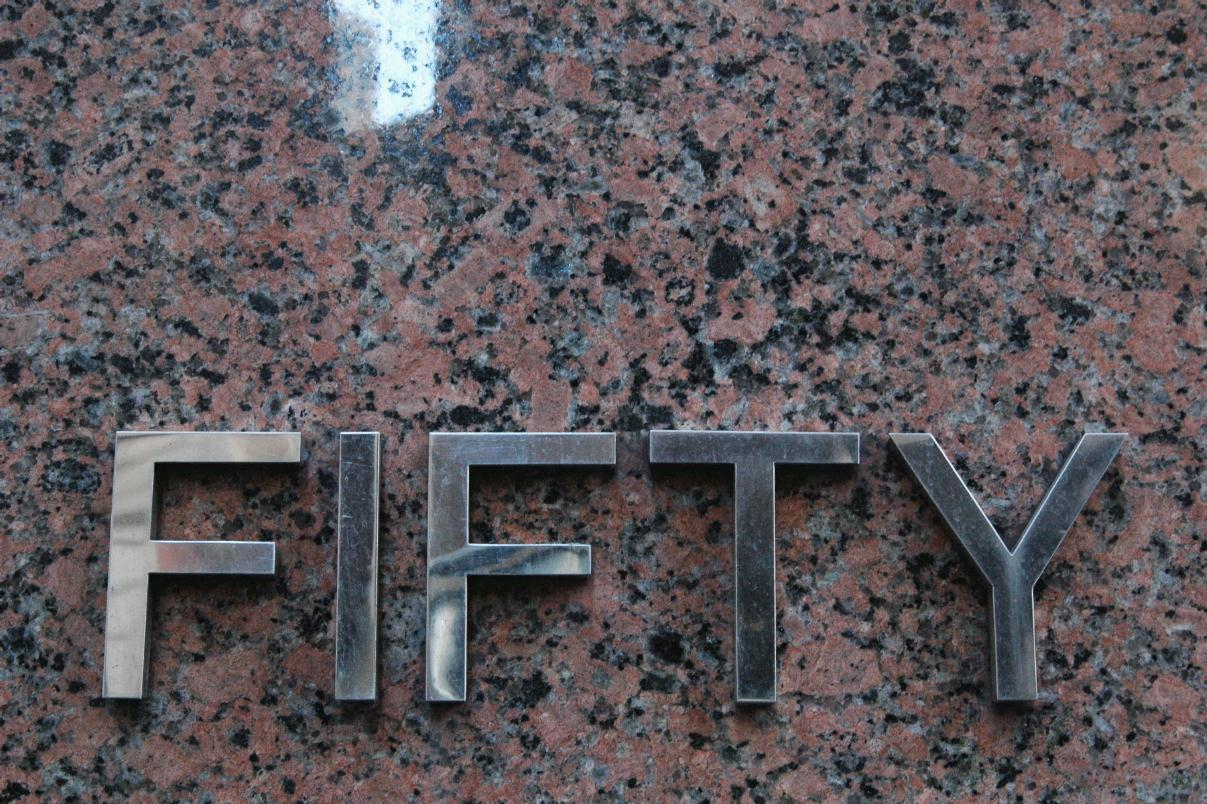Fifty, Number as a Word, as Found Art50 (fifty) is the natural number following 49 and preceding 51[from wikipedia]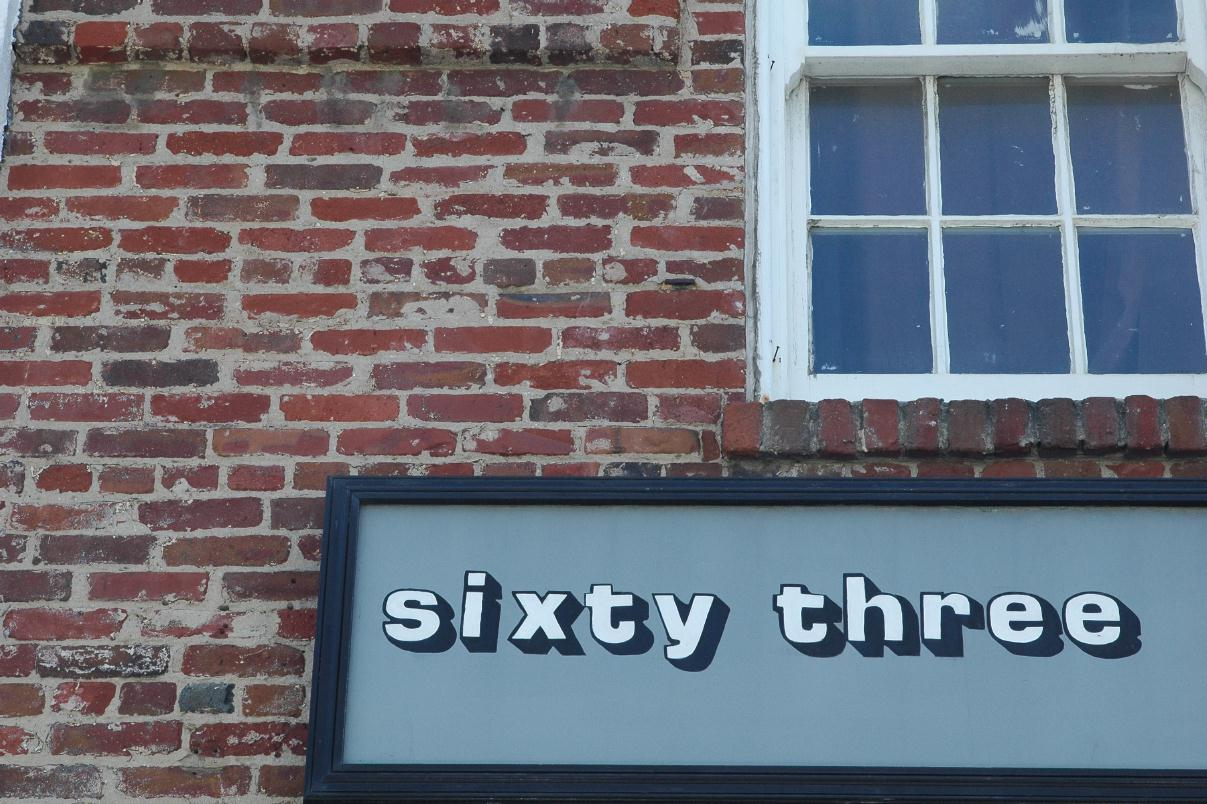Sixty-Three, Number as a Word, as Found Art63 (sixty-three) is a natural number following 62 and preceding 64.[from wikipedia]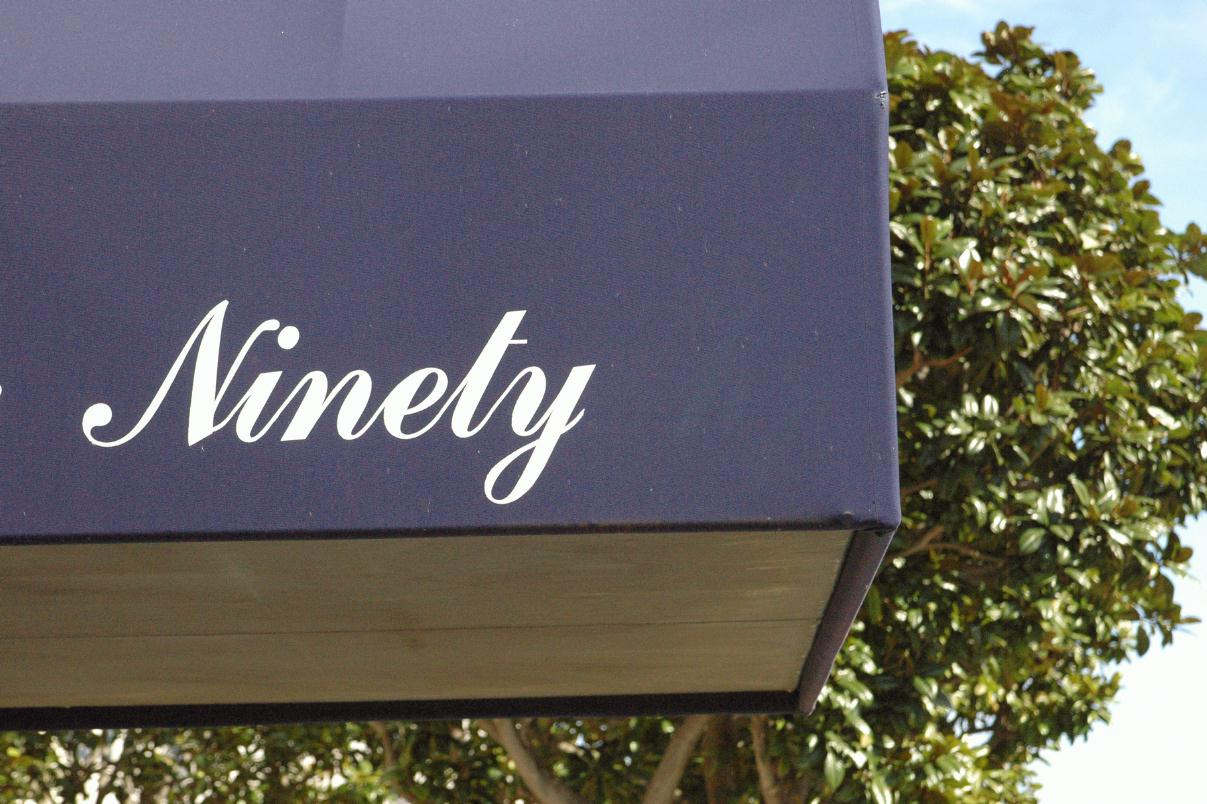Ninty, Number as a Word, as Found Art90 (ninety) is the natural number preceded by 89 and followed by 91.[from wikipedia]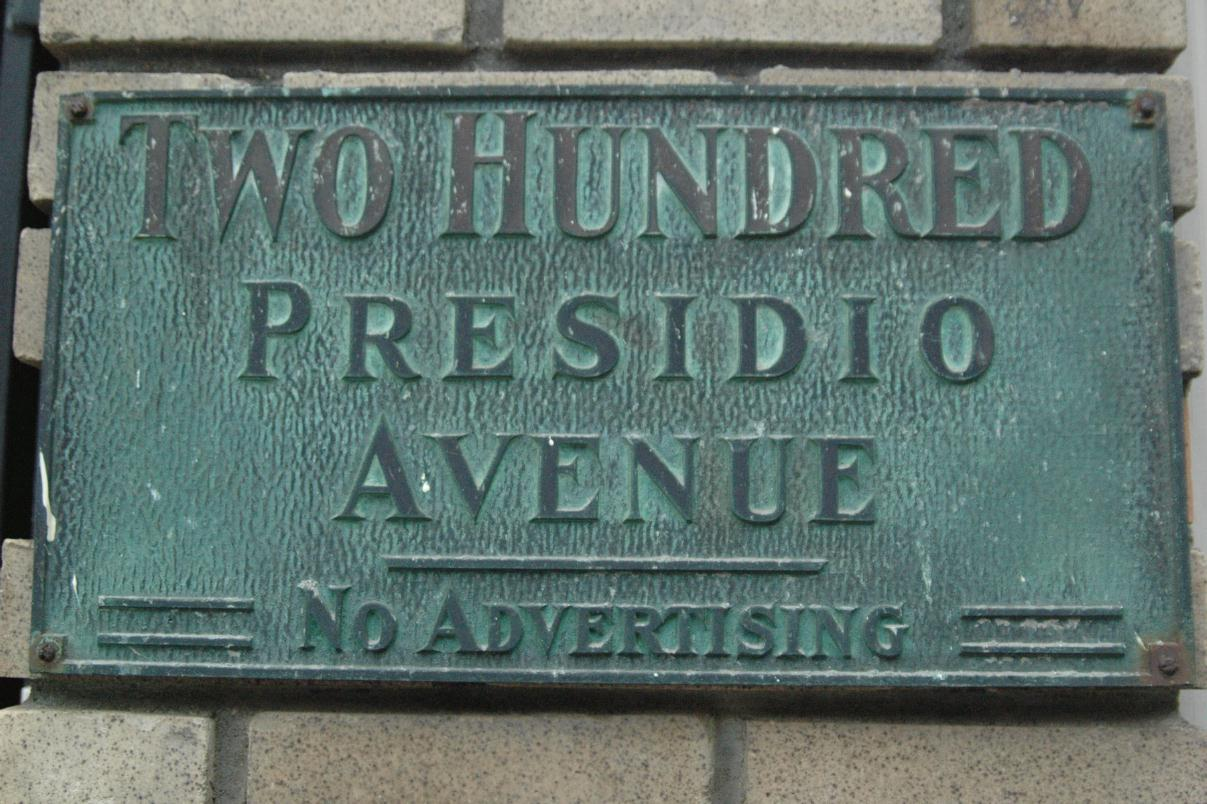Two-Hundred, Number as a Word, as Found Art200 (two hundred) is the natural number following 199 and preceding 201.[from wikipedia]

Subscribe to our mailing list for a Sunday summary of the week's stories.
* indicates required
Email Formathome • contact • topic guide • top 25 • photos • video • writing • blogs • upload • terms • privacy